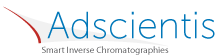## Surface Morphology

### Quick Start

Via the clever use of various branched probes, IGC lets you work out the morphology of your surface.

### Surface Morphology

Branched probe
Tmeasure °C
χT
Vgprobe
ΔGprobe
IMprobe

A set of IGC measurements with alkane probes gives their Vg values (specific retention volumes) which in turn are normalised values calculated from retention times.From these Vg values the free energy of absorption, ΔG can be calculated via:

ΔG = RTmln(Vg)

where R is the universal gas constant, Tm is the measurement temperature (converted from °C to K).

In addition the Vg value of a branched alkane is measured. Knowing the topology index χT of the branched alkane and given that by definition the topology of the linear alkanes is the same as their number of carbon atoms it is possible to calculate a morphology index IM as:

IM = VgBranched/VgLinear

Here VgLinear is the calculated Vg of the hypothetical linear hydrocarbon with the same χT value. This in turn is obtained from the linear plot of ΔG values of the linear hydrocarbons. It is easier to see things in the linear ΔG space than the non-linear Vg space.

For a smooth surface, so that the branched molecule can make the same contact as the linear equivalent, IM=1. If the surface is rough, the branched molecule makes less contact with the surface so has a lower Vg. So the smaller IM, the rougher the surface.

Enter Vg values for at least two alkanes along with the Vg for your probe molecule plus its χT selected from the following list of some of the branched alkanes (a fuller table has been published by Brendlé and Papirer1):

The data points and the least squares fit which defines the reference line and the theoretical value of VgLinear are shown.

1Eric Brendlé and Eugène Papirer, A New Topological Index for Molecular Probes Used in Inverse Gas Chromatography: 1. Method of Evaluation, Journal of Colloid and Interface Science, 1194, 207–216 (1997)The IGC apps are based on the inputs kindly provided by Dr Eric Brendlé of Adscientis who are specialists in IGC measurements.